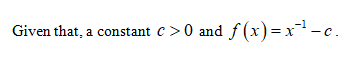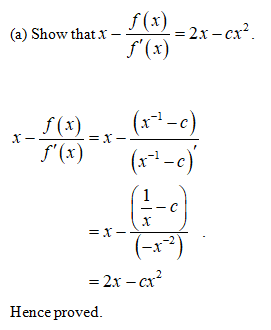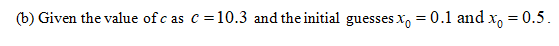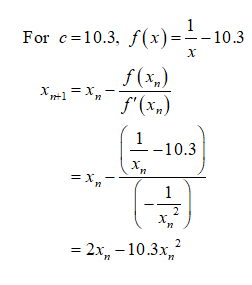# Newton’s Method can be used to compute reciprocals without performing division. Letc > 0 and set f (x) = x−1 −c.(a) Show that x − (f (x)/f' (x)) = 2x − cx2.(b) Calculate the first three iterates of Newton’s Method with c = 10.3 and the two initial guesses x0 = 0.1 and x0 = 0.5.(c) Explain graphically why x0 = 0.5 does not yield a sequence converging to 1/10.3.

Question
1 views

Newton’s Method can be used to compute reciprocals without performing division. Letc > 0 and set f (x) = x
−1 −c.
(a) Show that x − (f (x)/f' (x)) = 2x − cx2.
(b) Calculate the first three iterates of Newton’s Method with c = 10.3 and the two initial guesses x0 = 0.1 and x0 = 0.5.
(c) Explain graphically why x0 = 0.5 does not yield a sequence converging to 1/10.3.

check_circle

Step 1Step 2Step 3...

### Want to see the full answer?

See Solution

#### Want to see this answer and more?

Solutions are written by subject experts who are available 24/7. Questions are typically answered within 1 hour.*

See Solution
*Response times may vary by subject and question.
Tagged in